# On certain rational perfect numbers

József Sándor
Notes on Number Theory and Discrete Mathematics
Print ISSN 1310–5132, Online ISSN 2367–8275
Volume 28, 2022, Number 2, Pages 281–285
DOI: 10.7546/nntdm.2022.28.2.281-285

## Details

### Authors and affiliations

József SándorDepartment of Mathematics, Babeș-Bolyai University
Cluj-Napoca, Romania

### Abstract

We study equations of type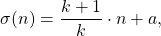where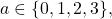where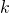and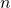are positive integers, while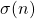denotes the sum of divisors of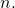### Keywords

• Sum of divisors
• Perfect numbers

• 11A25

### References

1. Sándor, J. (1989). On the composition of some arithmetic functions. Studia Universitatis Babeș-Bolyai, Mathematica, 34(1), 7–14.
2. Sándor, J. (2001). On even perfect and superperfect numbers. Notes on Number Theory and Discrete Mathematics, 7(1), 4–5.
3. Sándor, J. (2006). An extension of k-perfect numbers. Research Group in Mathematical Inequalities and Applications, 9(4), Article 4.
4. Sándor, J. (2008). On kp-perfect numbers. Matlap, 12(8), 297–298 (in Hungarian).
5. Sándor, J., & Atanassov, K. T. (2021). Arithmetic Functions. Nova Science Publishers, New York.
6. Sándor, J., Mitrinović, D. S., & Crstici, B. (2006). Handbook of Number Theory I. Springer, Dordrecht.